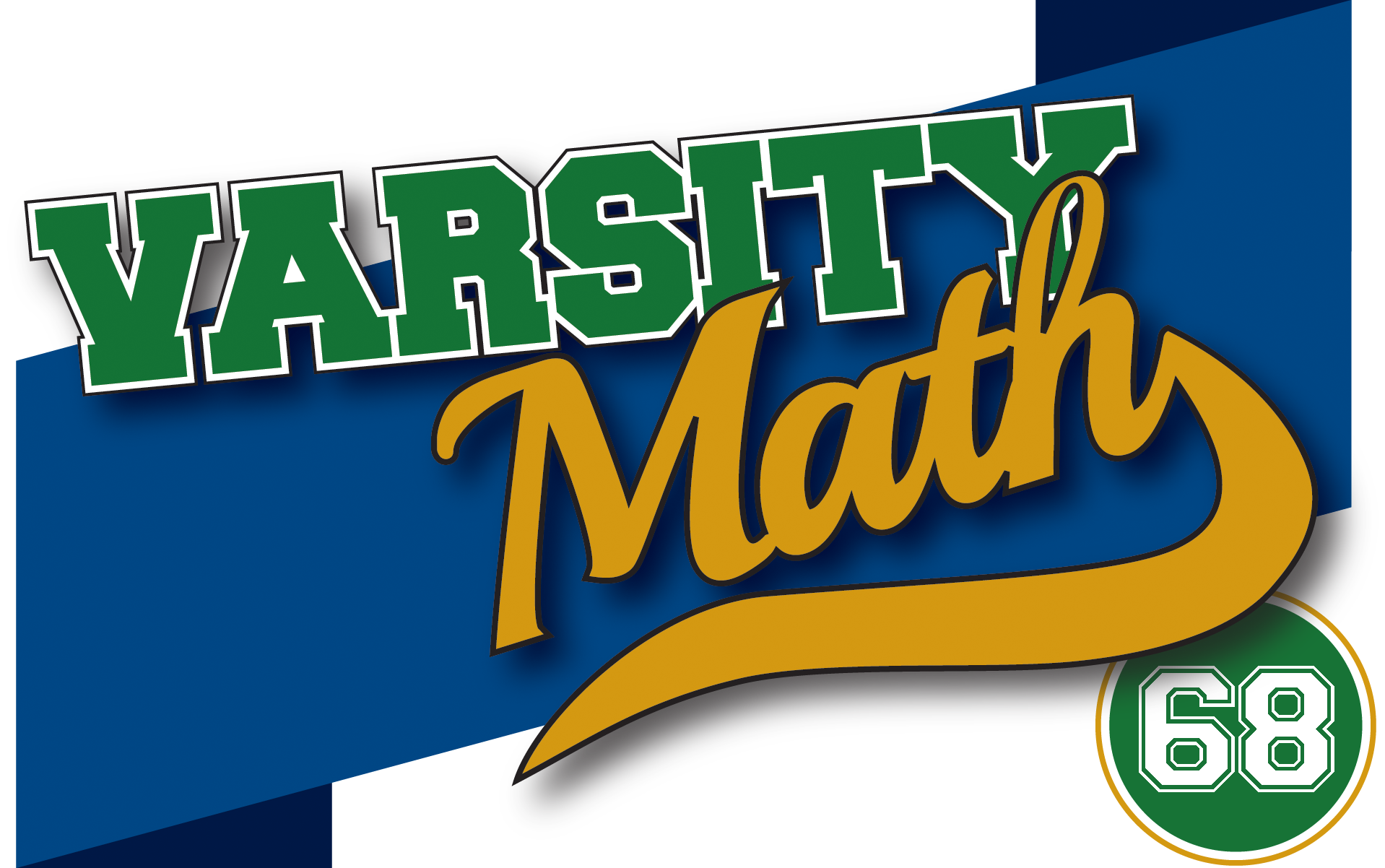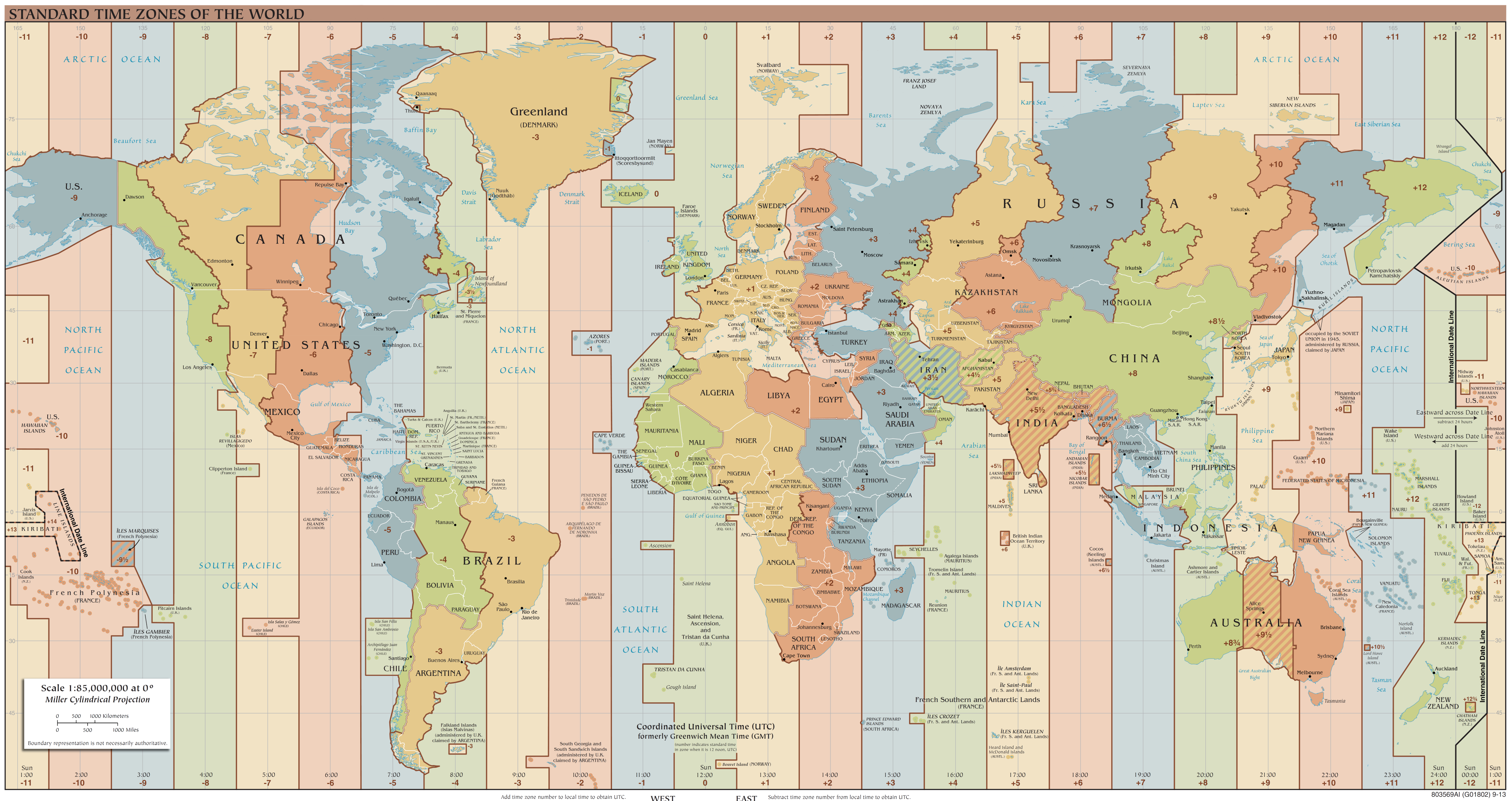## ________________

The fascination with circles among the Varsity Math crew continues, this time with one completely abstract and one very concrete problem.

## ________________

### Snug Circle

The length of the radius of circle b is six, and its center is at coordinates (0,6). Circle a is tangent both to circle b and to the line y=0; the two points of tangency are different. The radius of circle a is two.

What are the absolute values of the coordinates of the center of circle a?### Congruence Time

The Bicoastal Cafe displays two clocks, one on Los Angeles time and the other on New York time (three hours ahead). At any given time, the hour and minute hands on each clock form some angle, which should always be considered a non-negative number of degrees less than or equal to 180°.

What is the earliest time in the day in Los Angeles (reckoning the beginning of a day at the stroke of midnight there) that the angles formed by each of the clocks are equal?

## Solutions to week 67Card T. Let E be the center of circle a. A careful diagram like the one at the right seems to indicate that the line DA is tangent to circle a, but how can we be sure? Well, it would suffice to show that radius EA is perpendicular to line DA. To this end, let angle BEA have measure x degrees. Then, since triangle BEA is isosceles, angle BAE has measure 90 – x/2. Also, triangle BEC is congruent to BEA, so the measure of angle AEC is 2x. Since triangle CEA is isosceles, this means that angle CAE has measure 90 – x. Taking the difference of the two known angles at A, we see that angle BAC has measure x/2. But triangle DBA is congruent to triangle CBA, so the measure of angle BAD is also x/2. Adding up the three known angles at A, we see that the measure of angle EAD is 90°. Hence, line DA interesects circle a just one time, at A.Card 61. Since BF is parallel to CE, you can create a smaller rectangle by “erasing” quadrilateral BCEF and squashing the two remaining triangles together vertically. Since quadrilateral BCEF has area 1/7 that of rectangle ACDF, the resulting rectangle must have area 6/7 of rectangle ACDF. But it has the same length AF, so it must have height AB 6/7 of the height AC of ACDF.

## Recent Weeks

Links to all of the puzzles and solutions are on the Complete Varsity Math page.

Come back next week for answers and more puzzles.

[asciimathsf]